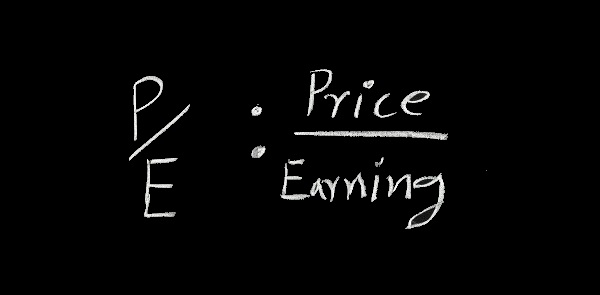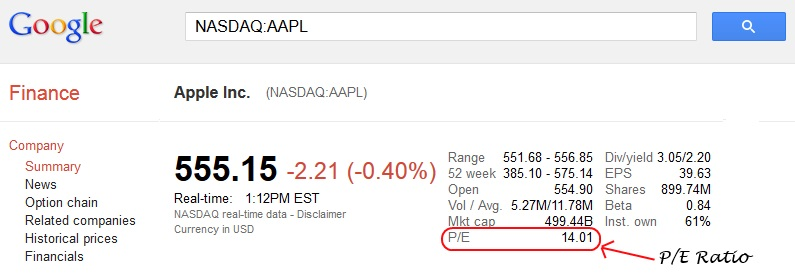Select Page

# PE Ratio: Introduction To The Price to Earnings RatioThe Price to Earnings ratio (P/E ratio) is probably the most commonly used financial ratios. Just about every financial website displays the P/E ratio as it is the most widely used investing metric for evaluating stocks.

The P/E ratio is considered a valuation metric for a company’s stock price. This ratio was made popular by famed investor Benjamin Graham and Warren Buffett, both of whom use this financial ratio as a quick way to determine if a stock is trading on an investment or speculative basis.

## How The PE Ratio is Calculated

The PE ratio is easy to calculate, it simply takes the stocks current share price and is divided by the latest earnings per share.

Calculated as: Share Price / Earnings per Share (EPS) = P/E RatioTypically, the P/E ratio is calculated using the most recent 12 month period of earnings, often called “trailing p/e.” It can also be calculated using the estimated net earnings over next 12 months, which is called “forward p/e.”

## P/E Ratio Example

Apple is currently trading at \$555 per share and had earnings of \$39 per share. Apple’s P/E ratio is 14 (555 / 39 = 14).

Most stock-quote systems such as Google Finance, Yahoo Finance or your online broker will automatically figure the price-to-earnings ratio for you. For example if you go to Google Finance to get a quote on a stock you can find out the P/E ratio, which is automatically displayed.## What is a Good P/E ratio?

A low P/E ratio is often thought as good and that a stock is trading at a great value. Many value investors use the P/E ratio to find stocks that are undervalued.

A low P/E may indicate the potential for strong future performance. Companies with low P/Es may be undervalued, or trading at a price lower than the company’s fundamentals merit. In that case, earnings may increase dramatically in future weeks and years.

However, just because a stock is trading at a low PE ratio doesn’t necessarily mean a stock is undervalued. Sometimes a lower PE ratio can signify that the company is having slower growth or the market expects the company not to do well and decrease earnings.

A high P/E ratio may signify that the company is overvalued, which means that eventually market forces will drive the price down. On the other hand, a higher P/E ratio could indicate that the company is expected to grow and do well, and earnings will increase over time, justifying the higher multiple.

Many companies that are growing at a fast rate will typically have a higher P/E ratio because higher premiums are given to faster growing companies. For example, Apple and Google once traded at very high p/e ratios as the stocks continued to double and triple in value.

For individual stocks, a good rule of thumb is you should compare a stock’s P/E with those of its competitors.

The P/E ratio range varies between sectors/indexes. For example, the small cap index will likely have a higher P/E ratio than the large cap index as the expected growth rate is higher.

The bottom line is that while PE ratio is a valuable tool, it doesn’t provide all the information you need to make an informed decision.

## PE Ratio Reference Guide

While there are various interpretations of the PE ratio, the table below is a guide of what the P/E ratio can signify.

P/E Ratio
N/A A P/E ratio that is n/a or is undefined is a company with no earnings. Companies with negative earnings (losses) have undefined PE ratios. For these companies a PE ratio can not be mathematically determined.
0–10 A P/E ratio in this range can mean that the stock is undervalued, or it could also mean the company’s earnings are to decline.
10–17 A P/E ratio in this range can mean the stock is trading at fair value.
17–25 A P/E ratio in this range can either mean the stock is overvalued or the company’s earnings have increased since the last earnings figure was published. The stock may also be a growth stock with earnings expected to increase in the future.
25+ A P/E ratio above this number can mean a company has very high expectations and is expected to have future growth in earnings, or this year’s earnings may be considered exceptionally low.